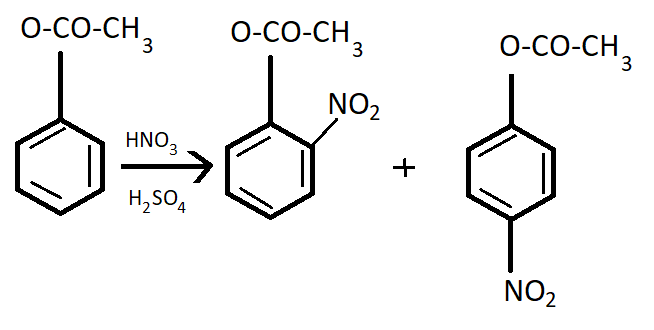QuestionAnswers

# Mononitration of phenyl methanoate.Verified
Hint: Formate whose IUPAC name is methanoate is the anion derived from formic acid. Its formula is chloroformate, $C{H_3}OCOCl$. The combined influence of —$OH$ and —$C{H_3}$ groups determines the position of the incoming group.
Phenyl methanoate is a compound having very less shelf- life. So, it is very unstable. Its formula is ${C_6}{H_5}OCOH$.It goes to the para position because the Benzene ring will be unstable if the $N{O_2}$group is attached anywhere else. It is an electron donating group and also an unstable compound at Meta or Ortho positions.
This process occurs in the presence of $HN{O_3}$ and ${H_2}S{O_4}$which is the nitrating mixture.